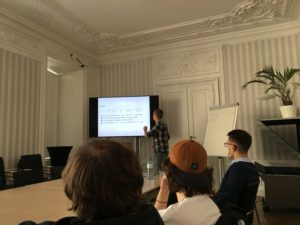Floragasse 7 – 5th floor, 1040 Vienna

# News

## Guest Lecture by Markus Hittmeir ‘A babystep-giantstep method for faster deterministic integer factorization’

Markus Hittmeir gave a lecture on parts of his PhD thesis on how to improve integer factorization.Abstract. The topic of this talk is the problem of computing the prime factorization of natural numbers. In practice, a large variety of probabilistic and heuristic methods is used for this task. However, none of these algorithms is e􏰀cient and the problem itself is assumed to be computationally hard. The difficulty of factoring large integers is fundamental for the security of severalcryptographicl systems, one of which is the public-key scheme RSA.

A more theoretical aspect of the integer factorization problem concerns deterministic algorithms and the rigorous analysis of their runtime complexity. In 1977, Volker Strassen presented such a method based on fast polynomial arithmetic techniques. The procedure computes the prime factorization of any natural number N in time O􏰃(N1/4), which has been state of the art for the last forty years. In this talk, we discuss the core ideas for improving the bound by a superpolynomial factor. The runtime complexity of our algorithm is of the form